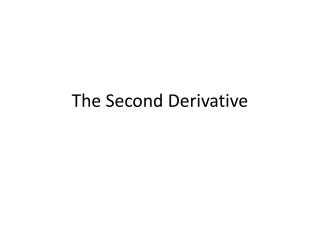DownloadDownload PresentationThe Second Derivative

# The Second Derivative

Télécharger la présentation## The Second Derivative

- - - - - - - - - - - - - - - - - - - - - - - - - - - E N D - - - - - - - - - - - - - - - - - - - - - - - - - - -
##### Presentation Transcript

1. The Second Derivative

2. Write a paragraph telling what information about the original function you can obtain when the graph of the derivative function is shown. Include as many details as possible.

3. GIVEN THE GRAPH OF f(x) CHOOSE THE GRAPH OF f ‘(x) In the next slides you will be presented (on the left) with the graph of the function, and on the right some choices for the derivative function. Choose the graph corresponding to the derivative function. • Give reason about your choice • For each of the graphs you did not choose give one reason why it was not chosen

4. GIVEN THE GRAPH OF f’(x) CHOOSE THE GRAPH OF f (x) In the next slides you will be presented (on the left) with the graph of the derivative function, and on the right some choices for the graph of the function (anti-derivative function). Choose the graph corresponding to the function. • Give reason about your choice • For each of the graphs you did not choose give one reason why it was not chosen

5. GIVEN THE GRAPH OF f’(x) CONSTRUCT A GRAPH OF f (x) Click on the link below to work on this activity. The graph in red is the derivative function of a function f(x). Start at the green point on the left hand of the window and use the information about the derivative of the function at that point to move the other points on the screen to reflect a point on the graph of f(x). You can check how accurate your graph is. Reconstructing a function from f ’

6. The Second Derivative Graphically you will find information about the graph of a function, its first and second derivative. Information about critical points and inflection points of the function will be obtained by looking at the graphs of the first and second derivatives.

7. Function and Its First Derivative

8. Second Derivative

9. First Derivative and Second Derivative The derivative function of the derivative function is called the secondderivative function

10. Higher Order Derivatives

11. Function and Its Second Derivative

12. Function, First, and Second Derivatives Local Max of f ’(x) Inflection Point of f(x) Concave up to concave down f “ changes from positive to negative

13. Function, First, and Second Derivatives f “ changes from negative to positive Inflection Point of f(x) Concave down to concave up Local min of f ’(x)

14. PRACTICE Select the correct answer in each case. On the intervals where a function is concave up, • The first derivative • Is positive/negative? • Is increasing/decreasing? • The second derivative • Is positive/negative? • Is increasing/decreasing?

15. Identifying f, f’, f’’ You will be presented with three graphs. They represent f, f ‘, f” . Determine which one is which. Give reasons for your choice